# Optics

The Aperture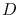of an optical system is (for radial-symmetry, i.e. circular, telescopes, which we will limit ourselves to consider) the diameter of its light-collecting area (i.e. its primary mirror or lens).
The Focal Length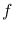of an optical system is expressed in metres and defines the image scale on the focal plane, or Focal Scale of the instrument, meaning that the angular distance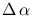between two directions on the sky is related to their physical distance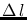when they are imaged on the focal plane by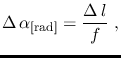which once "put in numbers" becomes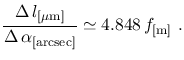Similarly, the solid angle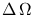spanned on the sky by a given sky region is related to the physical area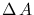spanned on the focal plane by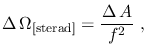which once "put in numbers" becomes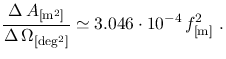The Focal Ratio (also referred to as f-number, f-ratio, Aperture Ratio and Relative Aperture and indicated as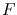or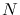) is defined as the ratio between the focal length and the aperture, e.g. by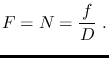It is often useful, and particularly so for simulation purposes, to describe the entire optical system of an astronomical instrument by means of a more or less simple mathematical function depending on a few parameters. In this context, it is also customary to describe the optical response of most "conventional" telescopes by means of some measure of the "size" of the instrument's beam.
A functional form often used for these purposes is the ideal diffraction pattern produced by a circular aperture, or Airy Function, whose exact analytical expression can be written as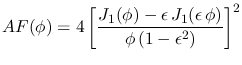(1)

where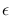is the telescope obstruction ratio (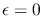in ideal systems),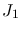is the Bessel J-function of order 1 and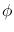is the dimensionless parameter related to the sky angle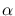by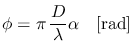For an ideal () system the Airy Function thus reduces to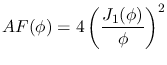(2)

and its Encircled Energy Function to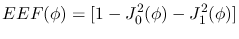(3)

The Airy Function and its Encircled Energy Function for an ideal system are tabulated in Table 1 for various values of(here expressed in units of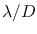).[]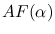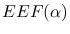0.0 1.000 0.0000 0.1 0.976 0.0244 0.2 0.905 0.0940 0.3 0.797 0.1989 0.4 0.664 0.3248 0.5 0.521 0.4559 0.6 0.381 0.5774 0.7 0.256 0.6785 0.8 0.154 0.7531 0.9 8.03E-2 0.8011 1.0 3.28E-2 0.8264 1.1 8.14E-3 0.8361 1.2 1.77E-4 0.8378 1.3 2.27E-3 0.8382 1.4 8.45E-3 0.8417 1.5 1.42E-2 0.8500

As mentioned, in practice a few "fiducial" values are used to characterize the extension of such an ideal diffraction pattern. An often-used proxy for an instrument's beam size is the Airy Disk, i.e. the first zero-brightness circle of the Airy Function. The angular diameter of the Airy Disk produced by a circular aperture of linear diameter D is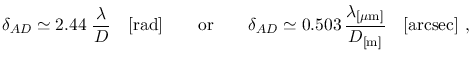so that the overall size of the "beam", or the solid angle encircled by the Airy Disk, is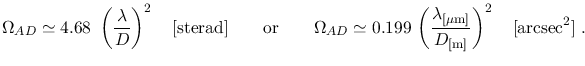Now the "full" Airy Disk above is a rather conservative (i.e. pessimistic) measure of the "size'"of an instrument's beam, which is why one often uses the Airy Disk FWHM (or more precisely the FWHM of the Airy Fucntion) for this purpose instead, whose value is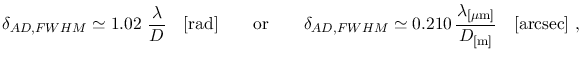so that the overall size of the "beam", or the solid angle encircled by the Airy Disk FWHM, is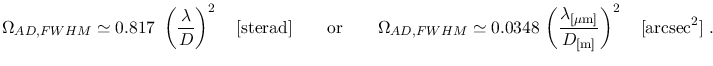The Focal Ratio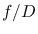of an optical system is the ratio between its focal length and aperture, whereas its reciprocal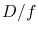is called Aperture Ratio.
For an ideal optical system (100 % reflectivity mirrors), the specific power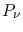hitting the whole detector when the system is exposed to a point source of specific brightness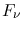is given by1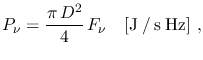(4)

or equivalently, expressed in photons, by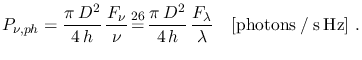(5)

Similarly, for an ideal optical system the specific power per unit solid angle hitting the detector when the system is exposed to a diffuse source of specific surface brightness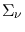is given by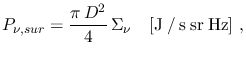(6)

or equivalently, expressed in photons, by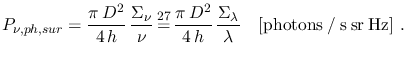(7)